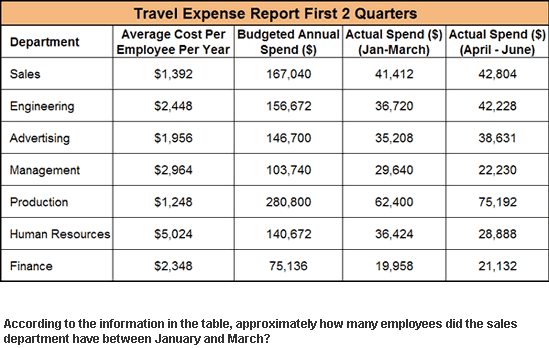# Subtle error in numeracy test question?They say the answer is 41412 / (1392 / 4) = 119.
I think you cannot say as they gave the average cost per employee per year so you know nothing about the cost per employee per year in the 1st Q so you cannot decide how many employees you got that quarter.

Last edited:

tiny-tim
Homework Helper
Hi Outlined!They say the answer is 41412 / (1392 / 4) = 119.
I think you cannot say as they gave the average cost per employee per year so you know nothing about the cost per employee per year in the 1st Q so you cannot decide how many employees you got that quarter.

But if you know the average cost per employee per year is 1392,

then the average cost per employee per quarter must be 1392/4.What is worrying you about that?Hi Outlined!But if you know the average cost per employee per year is 1392,

then the average cost per employee per quarter must be 1392/4.What is worrying you about that?thanks for your reply, however the employees who work in Q1 are not necessarily the same as the one who work in Q2, Q3 or Q4. So you have to be cautious drawing conclusion from that.

tiny-tim
Homework Helper
it's an average!what do you think averages are for??let me give you a counterexample.

p1 is the number of people who work in Q1
pr is the number of people who work in Q2, Q3 and Q4.

Than the average costs per employee per year equals

1/4 * 1/p1 * 41412 + 3/4 *1/ pr * (167040 - 41412) = 1392

Two free variables so c1 can be everything!!!

Last edited:
I see what you mean Outlined. The cost per employee could have been different in Q1. But since it's a test question you have to make enough assumptions to be able to give an answer. If you were awesome you'd state those assumptions, like "I assume the average cost per employee per year is the same as if the average is taken only over 1st quarter." Well there's probably a nicer way to put it :P

thanks for your reply, however with most of this kind of quesitons you do not have to make assumptions. In fact, some of this kind of exercises have the option (it is always multiple choice) "cannot say".

HallsofIvy
it's an average!what do you think averages are for??